ISEE Middle Level Math : Numbers and Operations

Example Questions

Example Question #41 : Numbers And Operations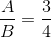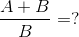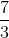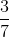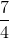Explanation:

By a proportion property, if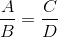, it follows that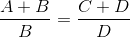.

Setting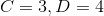if, then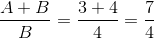.

Example Question #42 : Numbers And Operations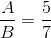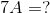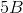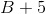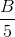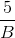Explanation:

The cross-products (the product of a numerator and the other denominator) of a proportion are equal, so, if, then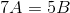.

Alice received $2.40 for her weekly allowance. If her favorite snacks cost 60¢ each, how many snacks can Alice buy this week? Possible Answers:Correct answer:Explanation: To solve: Divide the total amount of Alice's allowance ($2.40) by the price for each snack ($.60)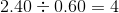Alice would be able to buy 4 snacks. Example Question #9 : How To Find The Part From The Whole Steve ate 20% of his pie on Monday. On Tuesday Steve ate 50% of the left-over pie. What percent of the pie is left? Possible Answers: 20% 40% 60% 10% 30% Correct answer: 40% Explanation: On Tuesday, Steve ate 50% (half) of the 80%, which is 40%. Steve has eaten 20% on Monday and 40% on Tuesday, so there is 40% left over. Example Question #10 : How To Find The Part From The Whole As a swim team member, Nicolas needs to train 90 hours a month. How many hours should Nicolas train each day of the 30-day month, in order to reach his goal? Possible Answers: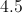Correct answer:Explanation: Divide 90 hours by 30 days in the month: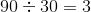Answer: Nicolas needs to train 3 hours a day. Example Question #11 : How To Find The Part From The Whole Brian found a motorcycle on sale for% off. If the original price of the motorcycle is$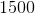, how much will Brian save?

$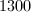$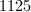$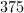$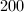$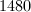Correct answer:$Explanation:

In order to find how much money Brian saved, we my first calculae% of $. We do this by multiplying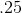by: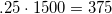Since we are looking for how much Brian saved and not how much he spent, our final answer is$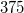.

Example Question #12 : How To Find The Part From The Whole

An eight slice pizza pie must be shared evenly among four friends. How many slices will each person get?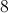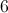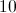Explanation:

Divide: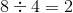Answer: Each person must get 2 slices.

Example Question #13 : How To Find The Part From The Whole

A teacher has sixty stickers to give out to her 15 students. How many stickers will each student receive?Explanation:

Divide: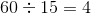Example Question #14 : How To Find The Part From The Whole

Sixteen balloons are to be shared evenly among four children. How many balloons will each child get?Explanation:

Divide: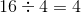balloons

Example Question #15 : How To Find The Part From The Whole

Seventy people need to make five equal lines. How many people will be on each line?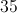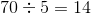people per line